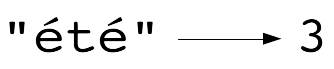# Programming-Idioms• Or search :

# Idiom #169 String length

Assign to the integer n the number of characters of the string s.
Make sure that multibyte characters are properly handled.
n can be different from the number of bytes of s.`n : Integer := s'Length;`
`(def n (.codePointCount s 0 (count s)))`
```IDENTIFICATION DIVISION.
PROGRAM-ID. length.
PROCEDURE DIVISION.
MOVE FUNCTION LENGTH(s) TO n
STOP RUN.```
```auto utf8len(std::string_view const& s) -> size_t
{
std::setlocale(LC_ALL, "en_US.utf8");

auto n = size_t{};
auto size = size_t{};
auto mb = std::mbstate_t{};

while(size < s.length())
{
size += mbrlen(s.data() + size, s.length() - size, &mb);
n += 1;
}

return n;
}```
`int n = s.Length;`
`import std.uni, std.range;`
`size_t n = s.byGrapheme.walkLength;`
`std.range;`
`size_t n = s.walkLength;`
`size_t n = s.length;`
`int n = s.length;`
`n = String.length s`
`N = string:length(S)`
`n = len(s)`
`import "unicode/utf8"`
`n := utf8.RuneCountInString(s)`
`n = length s`
`const n = s.length;`
`const n = [...s].length;`
`int n = s.length();`
`val n = s.length`
`(setf n (length s))`
`(length s)`
`local n = utf8.len(s)`
`@import Foundation;`
`NSUInteger n=s.length;`
`\$n = mb_strlen(\$s, 'UTF-8');`
`uses LazUtf8;`
`n := Utf8Length(s);`
`uses sysutils;`
`n := s.length;`
`uses LazUtf8;`
`n := length(s);`
`my \$n = length( \$s );`
`n = len(s)`
`n = s.size`
`n = s.length`
`let n = s.chars().count();`
`val n = s.length()`
`n := s size.`
```Dim n As Integer = s.Length
```

programming-idioms.org